International
Tables for
Crystallography
Volume F
Crystallography of biological macromolecules
Edited by M. G. Rossmann and E. Arnold

International Tables for Crystallography (2006). Vol. F, ch. 16.2, pp. 346-348   | 1 | 2 |

## Section 16.2.2. The maximum-entropy principle in a general context

G. Bricognea*

aLaboratory of Molecular Biology, Medical Research Council, Cambridge CB2 2QH, England
Correspondence e-mail: gb10@mrc-lmb.cam.ac.uk

### 16.2.2. The maximum-entropy principle in a general context

| top | pdf |

#### 16.2.2.1. Sources of random symbols and the notion of source entropy

| top | pdf |

Statistical communication theory uses as its basic modelling device a discrete source of random symbols, which at discrete times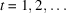, randomly emits a symbol' taken out of a finite alphabet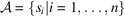. Sequences of such randomly produced symbols are called messages'.

An important numerical quantity associated with such a discrete source is its entropy per symbol H, which gives a measure of the amount of uncertainty involved in the choice of a symbol. Suppose that successive symbols are independent and that symbol i has probability. Then the general requirements that H should be a continuous function of the, should increase with increasing uncertainty, and should be additive for independent sources of uncertainty, suffice to define H uniquely as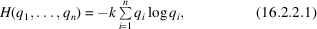where k is an arbitrary positive constant [Shannon & Weaver (1949), Appendix 2] whose value depends on the unit of entropy chosen. In the following we use a unit such that.

These definitions may be extended to the case where the alphabetis a continuous space endowed with a uniform measure μ: in this case the entropy per symbol is defined as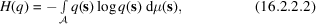where q is the probability density of the distribution of symbols with respect to measure μ.

#### 16.2.2.2. The meaning of entropy: Shannon's theorems

| top | pdf |

Two important theorems [Shannon & Weaver (1949), Appendix 3] provide a more intuitive grasp of the meaning and importance of entropy:

 (1) H is approximately the logarithm of the reciprocal probability of a typical long message, divided by the number of symbols in the message; and (2) H gives the rate of growth, with increasing message length, of the logarithm of the number of reasonably probable messages, regardless of the precise meaning given to the criterion of being reasonably probable'.

The entropy H of a source is thus a direct measure of the strength of the restrictions placed on the permissible messages by the distribution of probabilities over the symbols, lower entropy being synonymous with greater restrictions. In the two cases above, the maximum values of the entropy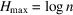and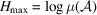are reached when all the symbols are equally probable, i.e. when q is a uniform probability distribution over the symbols. When this distribution is not uniform, the usage of the different symbols is biased away from this maximum freedom, and the entropy of the source is lower; by Shannon's theorem (2), the number of reasonably probable' messages of a given length emanating from the source decreases accordingly.

The quantity that measures most directly the strength of the restrictions introduced by the non-uniformity of q is the difference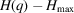, since the proportion of N-atom random structures which remain reasonably probable' in the ensemble of the corresponding source is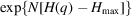. This difference may be written (using continuous rather than discrete distributions)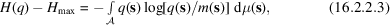where m(s) is the uniform distribution which is such that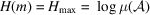.

#### 16.2.2.3. Jaynes' maximum-entropy principle

| top | pdf |

From the fundamental theorems just stated, which may be recognized as Gibbs' argument in a different guise, Jaynes' own maximum-entropy argument proceeds with striking lucidity and constructive simplicity, along the following lines:

 (1) experimental observation of, or data acquisition' on, a given system enables us to progress from an initial state of uncertainty to a state of lesser uncertainty about that system; (2) uncertainty reflects the existence of numerous possibilities of accounting for the available data, viewed as constraints, in terms of a physical model of the internal degrees of freedom of the system; (3) new data, viewed as new constraints, reduce the range of these possibilities; (4) conversely, any step in our treatment of the data that would further reduce that range of possibilities amounts to applying extra constraints (even if we do not know what they are) which are not warranted by the available data; (5) hence Jaynes's rule: The probability assignment over the range of possibilities [i.e. our picture of residual uncertainty] shall be the one with maximum entropy consistent with the available data, so as to remain maximally non-committal with respect to the missing data'.

The only requirement for this analysis to be applicable is that the ranges of possibilities' to which it refers should be representable (or well approximated) by ensembles of abstract messages emanating from a random source. The entropy to be maximized is then the entropy per symbol of that source.

The final form of the maximum-entropy criterion is thus that q(s) should be chosen so as to maximize, under the constraints expressing the knowledge of newly acquired data, its entropy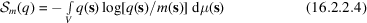relative to the `prior prejudice' m(s) which maximizes H in the absence of these data.

#### 16.2.2.4. Jaynes' maximum-entropy formalism

| top | pdf |

Jaynes (1957)solved the problem of explicitly determining such maximum-entropy distributions in the case of general linear constraints, using an analytical apparatus first exploited by Gibbs in statistical mechanics.

The maximum-entropy distribution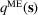, under the prior prejudice m(s), satisfying the linear constraint equations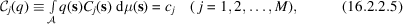where the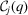are linear constraint functionals defined by given constraint functions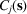, and theare given constraint values, is obtained by maximizing with respect to q the relative entropy defined by equation (16.2.2.4). An extra constraint is the normalization condition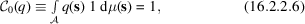to which it is convenient to give the label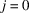, so that it can be handled together with the others by putting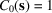,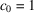.

By a standard variational argument, this constrained maximization is equivalent to the unconstrained maximization of the functional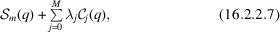where theare Lagrange multipliers whose values may be determined from the constraints. This new variational problem is readily solved: if q(s) is varied to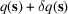, the resulting variations in the functionals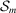and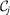will be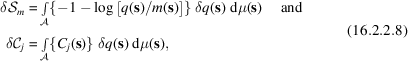respectively. If the variation of the functional (16.2.2.7)is to vanish for arbitrary variations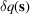, the integrand in the expression for that variation from (16.2.2.8)must vanish identically. Therefore the maximum-entropy density distributionsatisfies the relation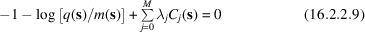and hence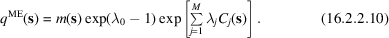It is convenient now to separate the multiplierassociated with the normalization constraint by putting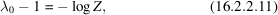where Z is a function of the other multipliers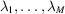. The final expression foris thus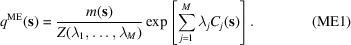The values of Z and ofmay now be determined by solving the initial constraint equations. The normalization condition demands that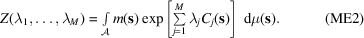The generic constraint equations (16.2.2.5)determineby the conditions that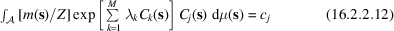for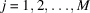. But, by Leibniz's rule of differentiation under the integral sign, these equations may be written in the compact form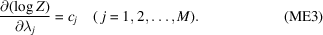Equations (ME1), (ME2) and (ME3) constitute the maximum-entropy equations.

The maximal value attained by the entropy is readily found: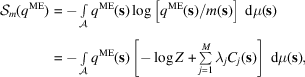i.e. using the constraint equations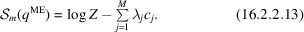The latter expression may be rewritten, by means of equations (ME3), as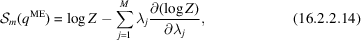which shows that, in their dependence on the λ's, the entropy and log Z are related by Legendre duality.

Jaynes' theory relates this maximal value of the entropy to the prior probability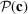of the vector c of simultaneous constraint values, i.e. to the size of the sub-ensemble of messages of length N that fulfil the constraints embodied in (16.2.2.5), relative to the size of the ensemble of messages of the same length when the source operates with the symbol probability distribution given by the prior prejudice m. Indeed, it is a straightforward consequence of Shannon's second theorem (Section 16.2.2)as expressed in equation (16.2.2.3)that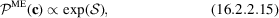where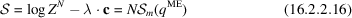is the total entropy for N symbols.

### References

Jaynes, E. T. (1957). Information theory and statistical mechanics. Phys. Rev. 106, 620–630.Google Scholar
Shannon, C. E. & Weaver, W. (1949). The mathematical theory of communication. Urbana: University of Illinois Press.Google Scholar